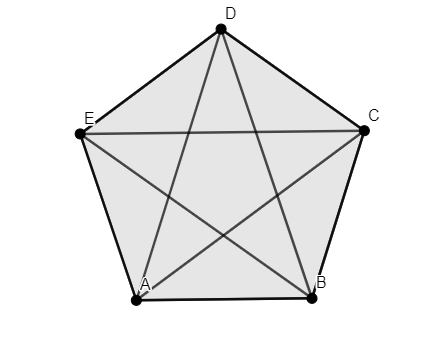Courses
Courses for Kids
Free study material
Free LIVE classes
MoreLIVE
Join Vedantu’s FREE Mastercalss

# Draw a rough sketch of a regular pentagon. The number of diagonals that can be drawn areA. 3B. 4C. 5D. None of the aboveVerified
334.5k+ views
Hint: To solve this type of problem first we have to draw pentagons which have 5 sides. Now applying the formula for the number of diagonals that can be formed. The formula is
Number of diagonals $=\dfrac{n\left( n-3 \right)}{2}$.

Step 1: Take 5 points like A, B, C, D, E.
Step 2: Join AB, BC, CD, DE, EA.
Step 3: Join AC, AD, BE, BD, CE.Now applying the formula for number of diagonals,
Number of diagonals$=\dfrac{n\left( n-3 \right)}{2}$ . . . . . . . . . . . . . . . . . . . . . . . . . . . . . . . . . . (a)
The number of sides of a pentagon is 5.
Now substituting the value of 5 in (a).
We get,
$=\dfrac{5\left( 5-3 \right)}{2}$
$=\dfrac{5\left( 2 \right)}{2}$
$=5$.
Therefore the number of diagonals that can be drawn is 5.
So, the correct answer is “Option C”.

Note: By applying the above formula we can find the number of diagonals for any polygon.Triangle is an exception to this rule, due to the shape of the triangle , it does not have any diagonals. Take care while drawing figures.
Last updated date: 22nd Sep 2023
Total views: 334.5k
Views today: 9.34k